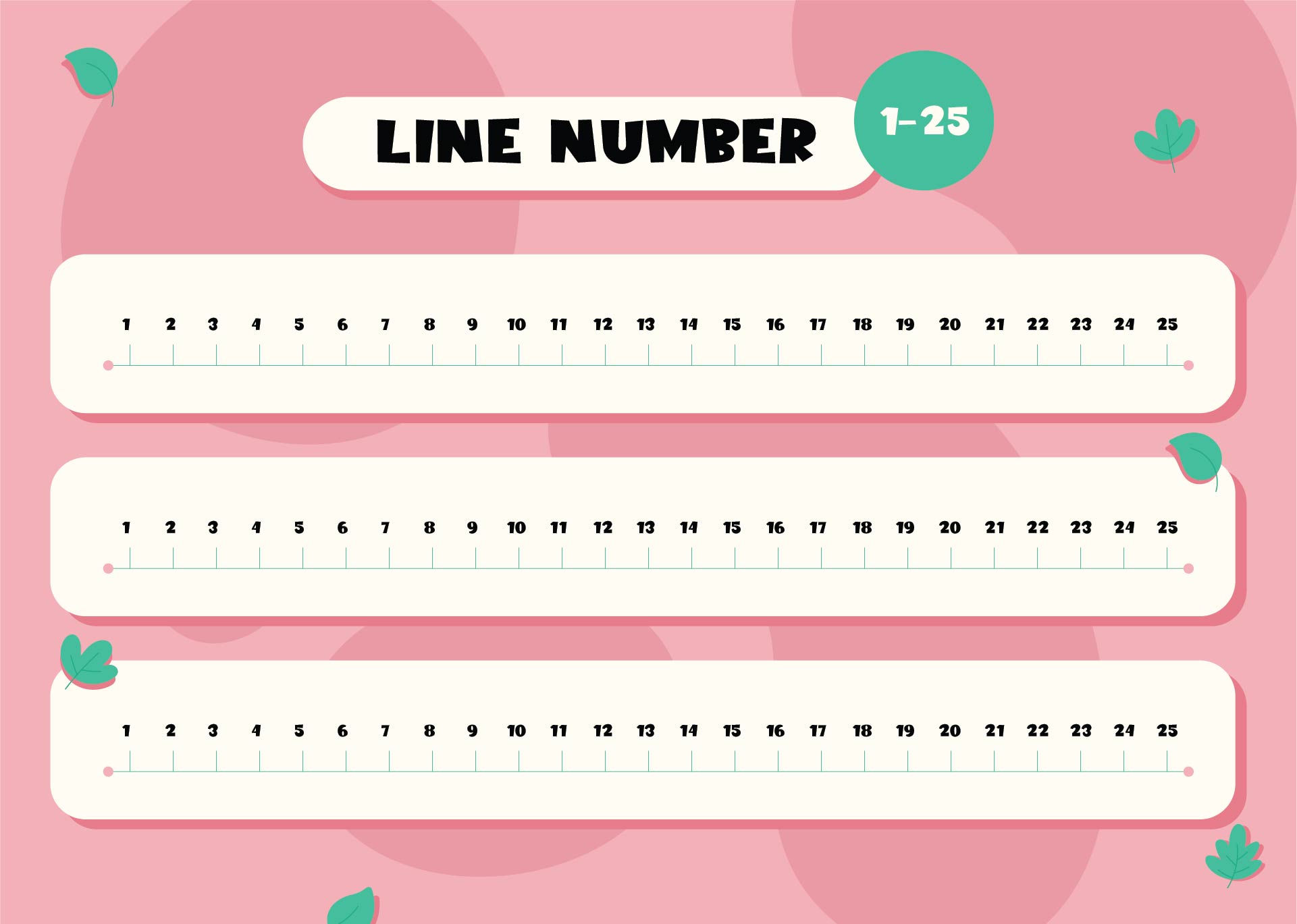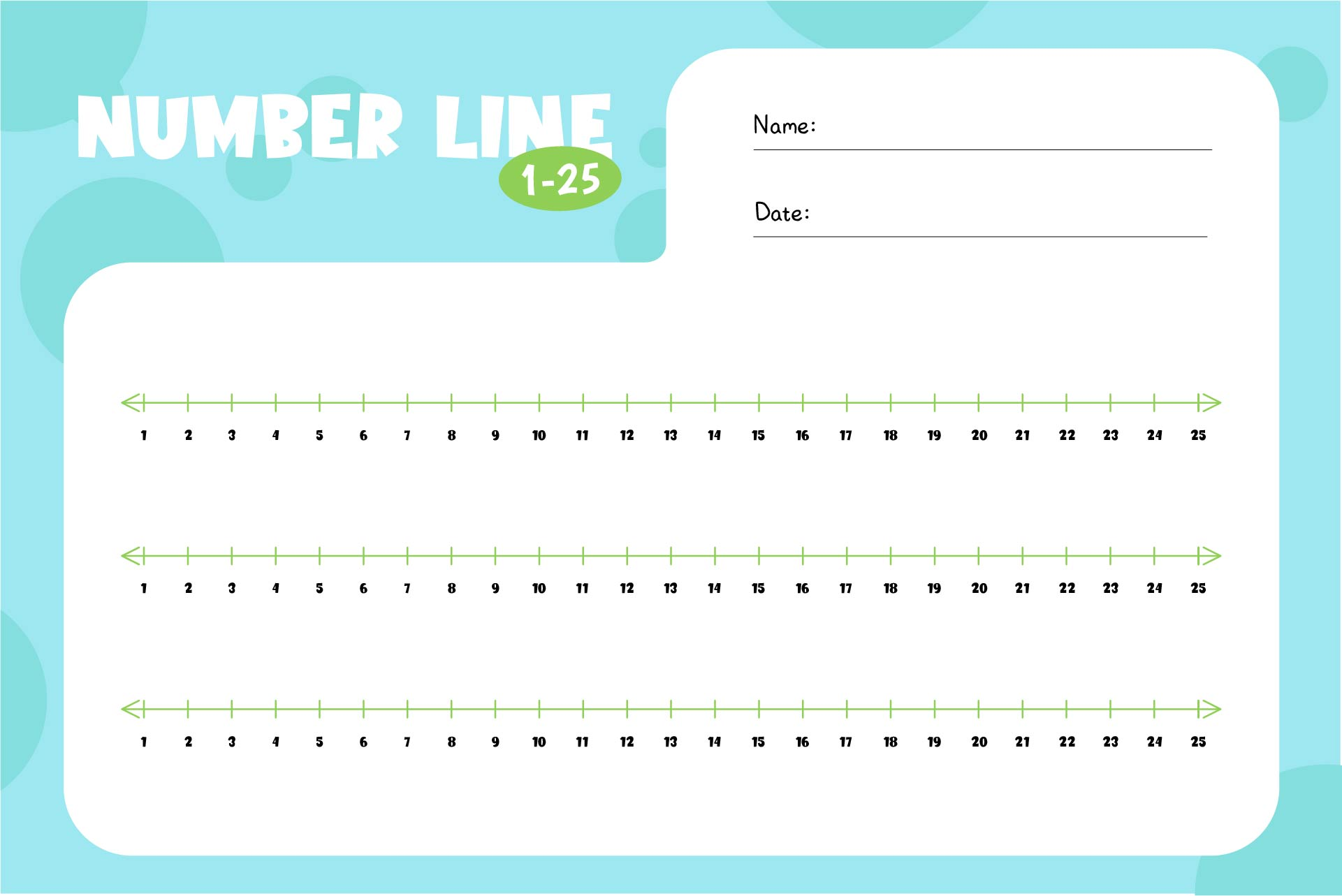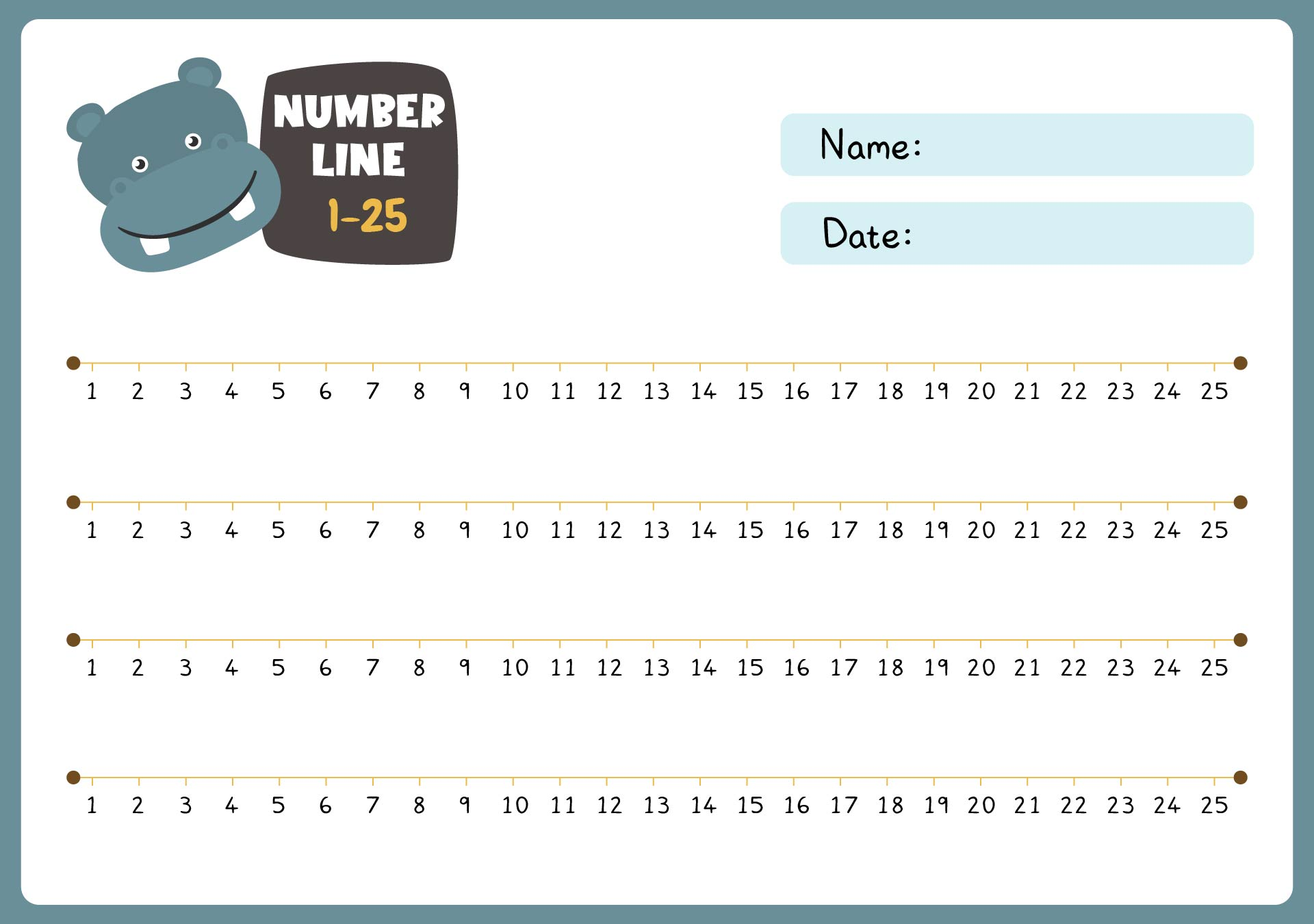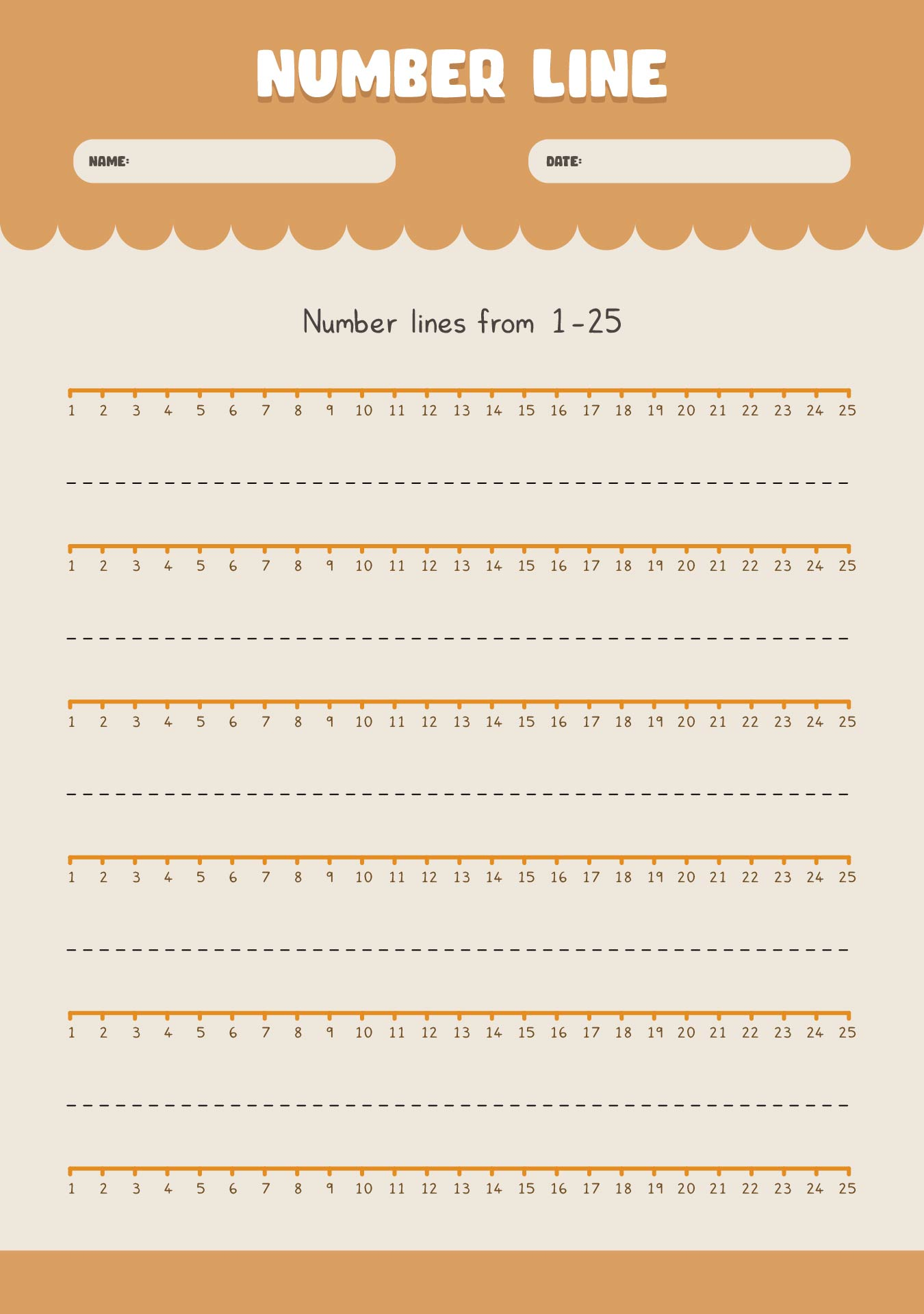# Printable Number Lines To 25Updated on Jan 19, 2023By Printablee Team### How do you solve number lines?

A number line is a visual representation of numbers that are arranged in order from least to greatest. Solving problems with number lines typically involves locating and identifying specific numbers on the line, as well as performing basic mathematical operations such as addition and subtraction. Here are a few steps to solving problems with number lines:

1. Understand the problem

Read the problem carefully and make sure you understand what is being asked.

1. Locate the numbers on the number line

Identify the numbers that are mentioned in the problem and locate them on the number line.

1. Perform the operation

Use the numbers on the number line to perform the mathematical operation that is being asked in the problem.

Label the answer on the number line, if the problem is asking for the location of a number.

Check your work to make sure the answer is correct. For example, if the problem is "What is 3 + 4?". Locate the number 3 on the number line. Count four spaces to the right of 3, you will land on 7, which is the answer.

In summary, number lines can be used to solve a variety of mathematical problems such as addition, subtraction, multiplication, and division. Number lines are a powerful tool for visualizing numbers and mathematical operations, and can be used to help solidify concepts for children and adults alike.

### What are number lines for kids?

Number lines are often used as a tool to help children understand basic mathematical concepts such as counting, addition, subtraction, and place value. When it comes to number lines for kids, they can take many forms from basic straight lines to more complex, interactive lines with movable pieces or markers.

They can be used with many different types of manipulatives, such as counting bears or blocks, to help children understand the relationship between numbers and quantities. Number lines can be used in a variety of ways to help children learn numbers, such as:

Counting. Kids can physically place manipulatives such as counting bears or blocks on the number line and count them as they move along the line.

Addition and subtraction. Children were allowed to use the number line to visualize the process of adding and subtracting numbers.

Number recognition. They can practice recognizing and identifying numbers using the number line.

Number sequencing. Practice putting numbers in numerical order is also part of the ability they can work with the number line.

Place Value. The number line gives kids’ the ability to understand the value of digits in numbers.

Comparing numbers. Number lines give clear visualization for kids to compare numbers and understand which one is greater or smaller.

Estimation. Estimating numbers and understanding the concept of rounding are also part of the benefits of using the number line.### Can I use board games to teach numbers?

If you are planning to set an engaging experience for kids in learning numbers, then using board games could be a great way to try. It can be an effective tool to use in a number-teaching setting. Here are the benefits of board games for kids:

Board games provide a fun and interactive way for children to learn numbers, as they physically manipulate objects such as game pieces or cards.

It can hold children's attention and keep them engaged in the learning process, which can help them retain the information better.

Playing board games allows children to practice and reinforce the numbers they have learned in a fun and non-threatening way.

Many board games require players to work together or take turns, which can help kids learn social skills and teamwork.

Board games can be adapted to different skill levels, so they can be used to teach numbers to children of different ages and abilities.

It allows kids to develop number sense, which is the ability to understand and use numbers in a flexible and efficient way.

### What are the basic number line skills?

After knowing the benefits of board games in terms of number learning, you might decide to have one in your classroom. Here are a couple of examples for you to try on!

1. Games like Chutes and Ladders or Snakes and Ladders can be used to let the kids master basic counting skills.
2. The Sum Swamp or Mathline are perfect for basic addition and subtraction skills.
3. When it comes to multiplication and division games, you can go with Multiplication War or Division Derby.
4. Place Value Pirates and Base Ten Battleship would be great to teach about placing the value and base 10 numbering system.
5. Fraction Frenzy or Fraction Flippers are such great board games to learn fractions.
6. Number Line Hop and Number Line Race would make the kids master number lines and number sense.
7. Number Match and Number Memory use number cards that can help the kids recognize, match and identify numbers.